# Average

Average of 7 numbers is 65. What is its sum?

Result

s =  455

#### Solution:Leave us a comment of example and its solution (i.e. if it is still somewhat unclear...):Be the first to comment!#### To solve this example are needed these knowledge from mathematics:

Looking for help with calculating arithmetic mean? Looking for a statistical calculator?

## Next similar examples:

1. AverageThe arithmetic mean of the two numbers is 71.7. One number is 5. Calculate the second number.
2. The squareThe square root of 25 times the square root of 81 is what number?
3. Watching TVOne evening 2/3 students watch TV. Of those students, 3/8 watched a reality show. Of the students that watched the show, 1/4 of them recorded it. What fraction of the students watched and recorded reality tv.
4. Double percent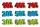What is 80% of 60% of 2800?
5. Number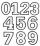Which number is 17 times larger than the number 6?
6. ClassIn 7.C clss are 10 girls and 20 boys. Yesterday was missing 20% of girls and 50% boys. What percentage of students missing?
7. Percentages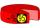Expressed as a percentage:
8. Equivalent fractionsAre these two fractions equivalent -4/9 and 11/15?
9. MultiplesFind all multiples of 10 that are larger than 136 and smaller than 214.
10. MidpointsTriangle whose sides are midpoints of sides of triangle ABC has a perimeter 45. How long is perimeter of triangle ABC?
11. Rolls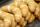Mom every day buys 6 rolls. On Friday buys 2 times as much. How many rolls buys on Friday?
12. Pins 2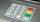how many different possible 4 digits pins can be found on the 10-digit keypad?
13. EmployeesOf all 360 employees, there are 11/12 women. How many men work in a company?
14. Coal mine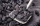The monthly plan of 17,000 tons of coal exceeded the mine by 1/25. How many tonnes of coal have been harvested from the mine above plan?Combine like terms 4c+c-7cWhich obtuse angle is creating clocks at 17:00?How many seeds germinated from 1000 pcs, when 23% no emergence?